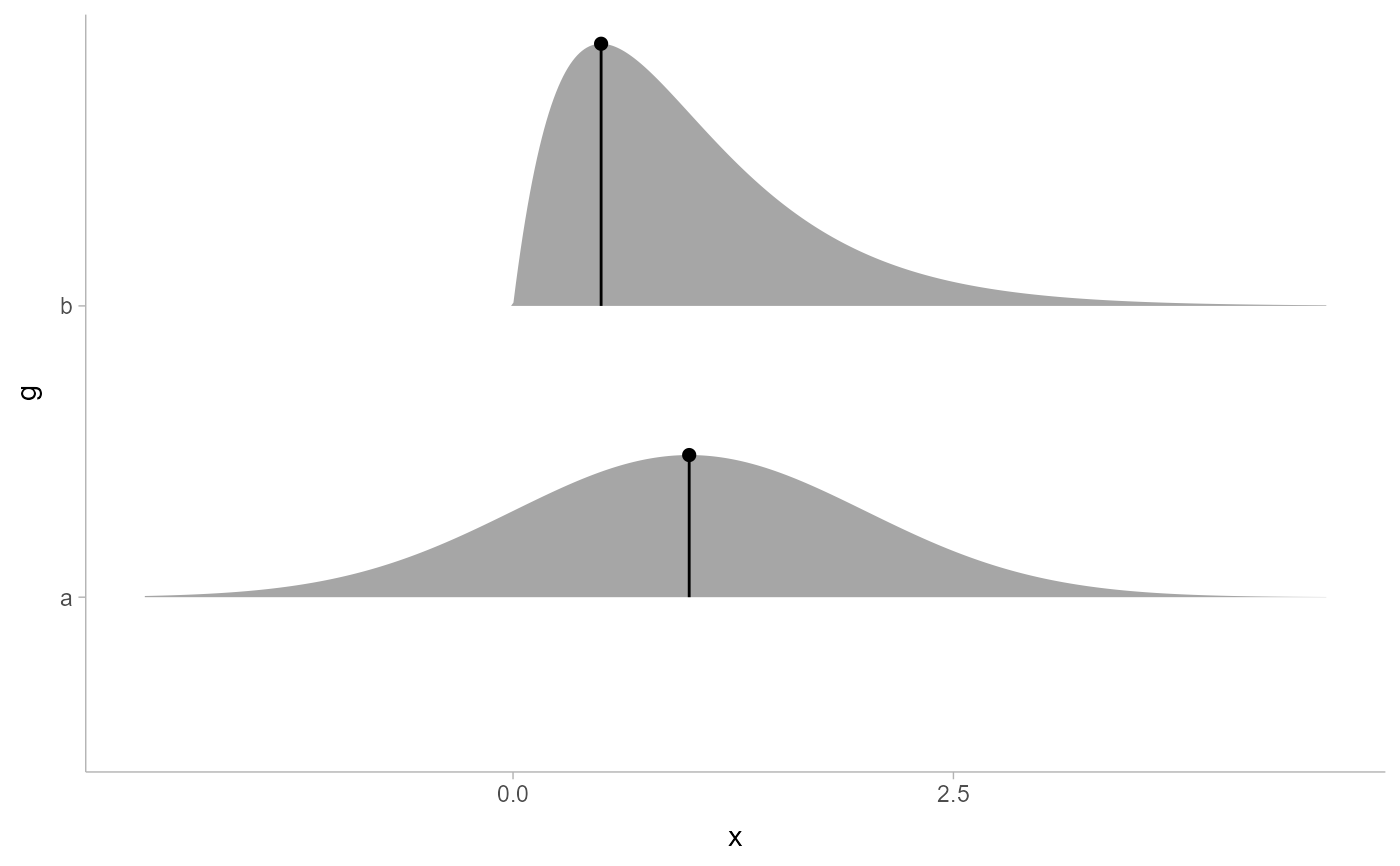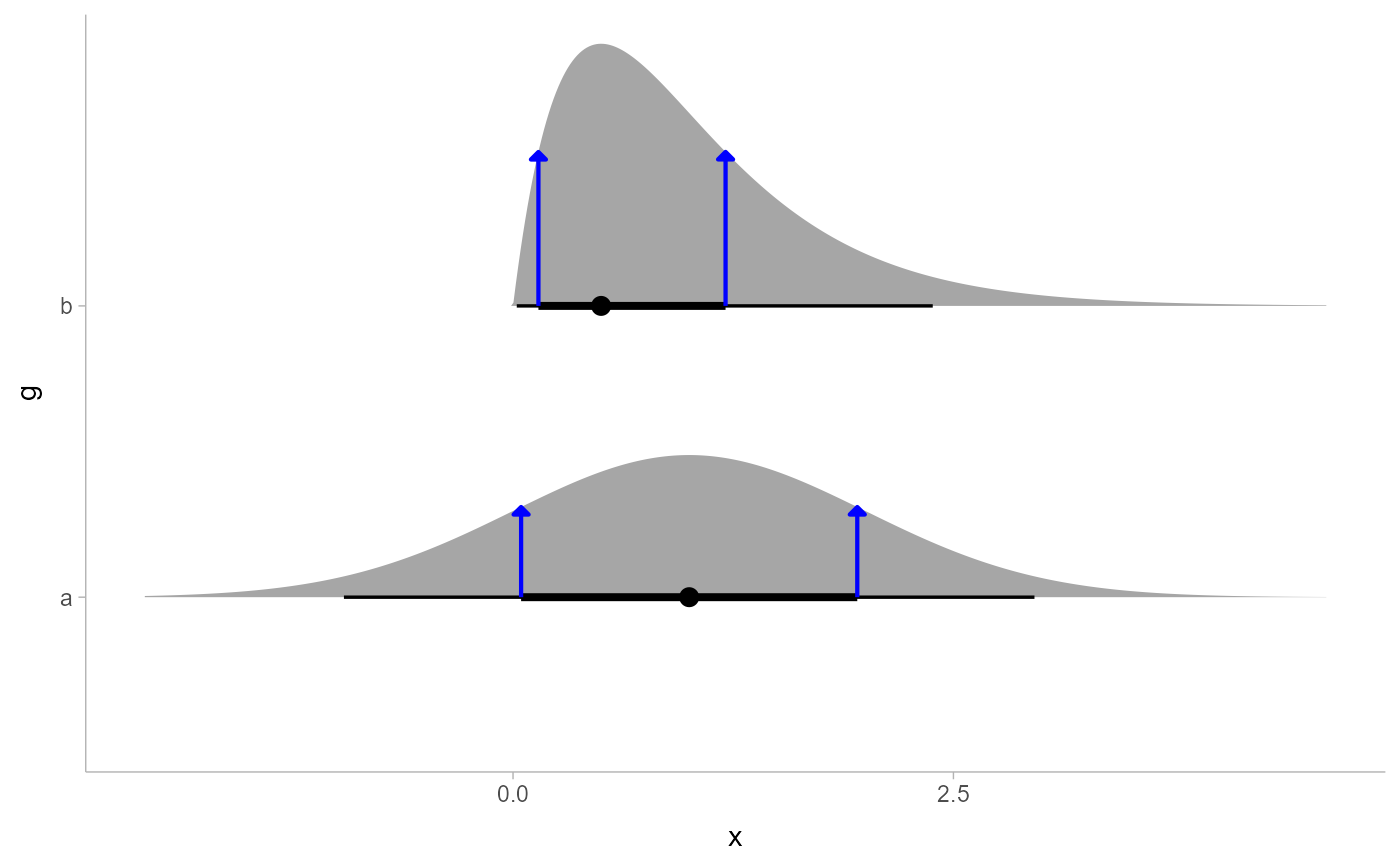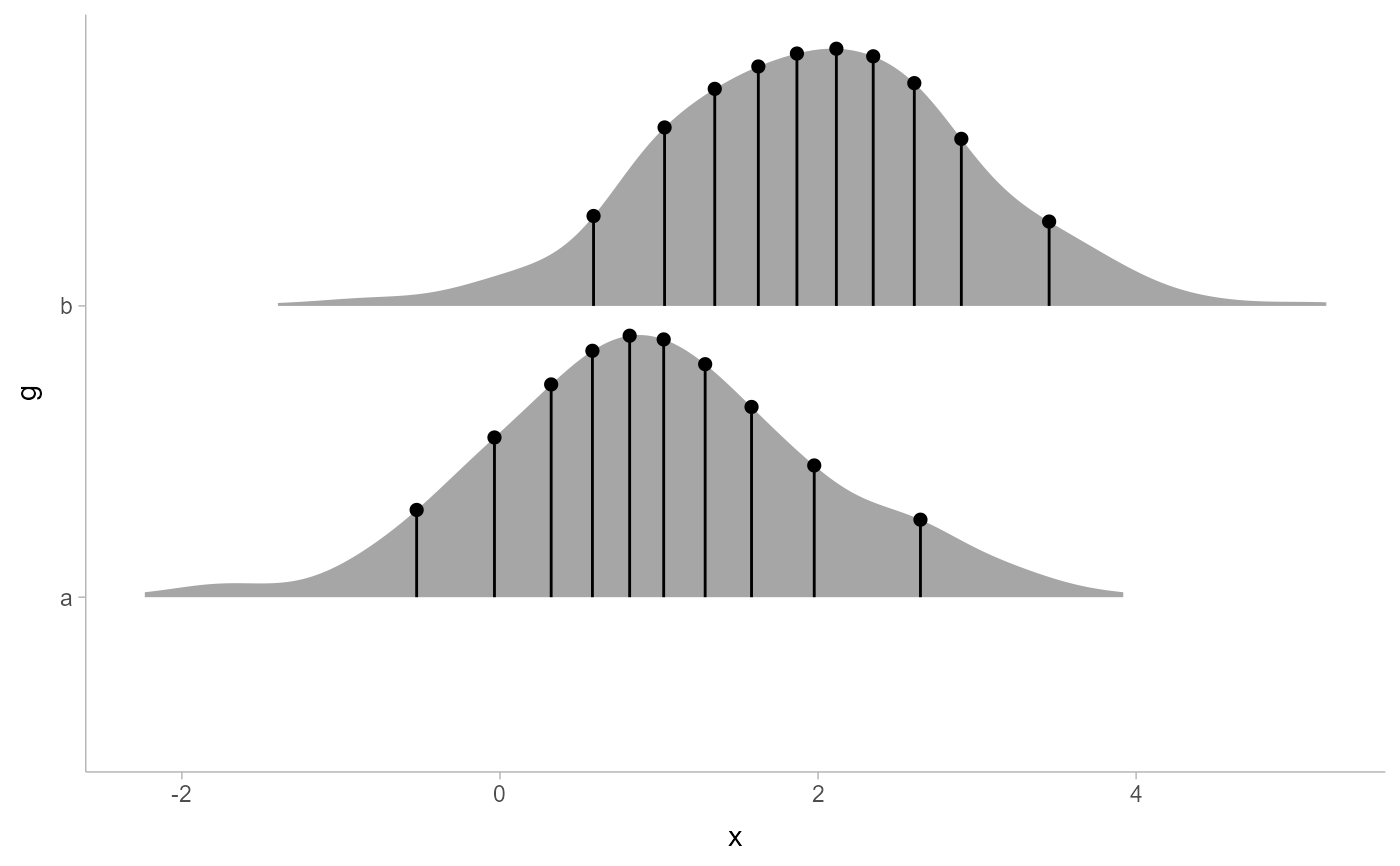Stat for drawing "spikes" (optionally with points on them) at specific points on a distribution (numerical or determined as a function of the distribution), intended for annotating stat_slabinterval() geometries.

stat_spike(
mapping = NULL,
data = NULL,
geom = "spike",
position = "identity",
...,
at = "median",
p_limits = c(NA, NA),
density = "bounded",
trim = TRUE,
expand = FALSE,
breaks = "Sturges",
align = "none",
outline_bars = FALSE,
slab_type = NULL,
limits = NULL,
n = 501,
orientation = NA,
na.rm = FALSE,
show.legend = NA,
inherit.aes = TRUE
)

## Arguments

mapping

Set of aesthetic mappings created by aes(). If specified and inherit.aes = TRUE (the default), it is combined with the default mapping at the top level of the plot. You must supply mapping if there is no plot mapping.

data

The data to be displayed in this layer. There are three options:

If NULL, the default, the data is inherited from the plot data as specified in the call to ggplot().

A data.frame, or other object, will override the plot data. All objects will be fortified to produce a data frame. See fortify() for which variables will be created.

A function will be called with a single argument, the plot data. The return value must be a data.frame, and will be used as the layer data. A function can be created from a formula (e.g. ~ head(.x, 10)).

geom

Use to override the default connection between stat_spike() and geom_spike()

position

Position adjustment, either as a string, or the result of a call to a position adjustment function. Setting this equal to "dodge" (position_dodge()) or "dodgejust" (position_dodgejust()) can be useful if you have overlapping geometries.

...

Other arguments passed to layer(). These are often aesthetics, used to set an aesthetic to a fixed value, like colour = "red" or linewidth = 3 (see Aesthetics, below). They may also be parameters to the paired geom/stat. When paired with the default geom, geom_spike(), these include:

arrow

grid::arrow() giving the arrow heads to use on the spike, or NULL for no arrows.

normalize

How to normalize heights of functions input to the thickness aesthetic. One of:

• "all": normalize so that the maximum height across all data is 1.

• "panels": normalize within panels so that the maximum height in each panel is 1.

• "xy": normalize within the x/y axis opposite the orientation of this geom so that the maximum height at each value of the opposite axis is 1.

• "groups": normalize within values of the opposite axis and within each group so that the maximum height in each group is 1.

• "none": values are taken as is with no normalization (this should probably only be used with functions whose values are in [0,1], such as CDFs).

at

The points at which to evaluate the PDF and CDF of the distribution. One of:

• numeric vector: points to evaluate the PDF and CDF of the distributions at.

• function or string: function (or name of a function) which, when applied on a distribution-like object (e.g. a distributional object or a posterior::rvar()), returns a vector of values to evaluate the distribution functions at.

• a list where each element is any of the above (e.g. a numeric, function, or name of a function): the evaluation points determined by each element of the list are concatenated together. This means, e.g., c(0, median, qi) would add a spike at 0, the median, and the endpoints of the qi of the distribution.

p_limits

Probability limits (as a vector of size 2) used to determine the lower and upper limits of theoretical distributions (distributions from samples ignore this parameter and determine their limits based on the limits of the sample). E.g., if this is c(.001, .999), then a slab is drawn for the distribution from the quantile at p = .001 to the quantile at p = .999. If the lower (respectively upper) limit is NA, then the lower (upper) limit will be the minimum (maximum) of the distribution's support if it is finite, and 0.001 (0.999) if it is not finite. E.g., if p_limits is c(NA, NA), on a gamma distribution the effective value of p_limits would be c(0, .999) since the gamma distribution is defined on (0, Inf); whereas on a normal distribution it would be equivalent to c(.001, .999) since the normal distribution is defined on (-Inf, Inf).

density

Density estimator for sample data. One of:

• A function which takes a numeric vector and returns a list with elements x (giving grid points for the density estimator) and y (the corresponding densities). ggdist provides a family of functions following this format, including density_unbounded() and density_bounded(). This format is also compatible with stats::density().

• A string giving the suffix of a function name that starts with "density_"; e.g. "bounded" for [density_bounded()], "unbounded" for [density_unbounded()], or "histogram" for density_histogram(). Defaults to "bounded", i.e. density_bounded(), which estimates the bounds from the data and then uses a bounded density estimator based on the reflection method.

Passed to density: the bandwidth for the density estimator for sample data is adjusted by multiplying it by this value. See e.g. density_bounded() for more information.

trim

For sample data, should the density estimate be trimmed to the range of the data? Passed on to the density estimator; see the density parameter. Default TRUE.

expand

For sample data, should the slab be expanded to the limits of the scale? Default FALSE. Can be length two to control expansion to the lower and upper limit respectively.

breaks

Determines the breakpoints defining bins. Similar to (but not exactly the same as) the breaks argument to graphics::hist(). One of:

• A scalar (length-1) numeric giving the number of bins

• A vector numeric giving the breakpoints between histogram bins

• A function taking x and weights and returning either the number of bins or a vector of breakpoints

• A string giving the suffix of a function that starts with "breaks_". ggdist provides weighted implementations of the "Sturges", "Scott", and "FD" break-finding algorithms from graphics::hist(), as well as breaks_fixed() for manually setting the bin width. See breaks.

For example, breaks = "Sturges" will use the breaks_Sturges() algorithm, breaks = 9 will create 9 bins, and breaks = breaks_fixed(width = 1) will set the bin width to 1.

align

Determines how to align the breakpoints defining bins. One of:

• A scalar (length-1) numeric giving an offset that is subtracted from the breaks. The offset must be between 0 and the bin width.

• A function taking a sorted vector of breaks (bin edges) and returning an offset to subtract from the breaks.

• A string giving the suffix of a function that starts with "align_" used to determine the alignment, such as align_none(), align_boundary(), or align_center().

For example, align = "none" will provide no alignment, align = align_center(at = 0) will center a bin on 0, and align = align_boundary(at = 0) will align a bin edge on 0.

outline_bars

For sample data (if density is "histogram") and for discrete analytical distributions (whose slabs are drawn as histograms), determines if outlines in between the bars are drawn when the slab_color aesthetic is used. If FALSE (the default), the outline is drawn only along the tops of the bars; if TRUE, outlines in between bars are also drawn. See density_histogram().

slab_type

(deprecated) The type of slab function to calculate: probability density (or mass) function ("pdf"), cumulative distribution function ("cdf"), or complementary CDF ("ccdf"). Instead of using slab_type to change f and then mapping f onto an aesthetic, it is now recommended to simply map the corresponding computed variable (e.g. pdf, cdf, or 1 - cdf) directly onto the desired aesthetic.

limits

Manually-specified limits for the slab, as a vector of length two. These limits are combined with those computed based on p_limits as well as the limits defined by the scales of the plot to determine the limits used to draw the slab functions: these limits specify the maximal limits; i.e., if specified, the limits will not be wider than these (but may be narrower). Use NA to leave a limit alone; e.g. limits = c(0, NA) will ensure that the lower limit does not go below 0, but let the upper limit be determined by either p_limits or the scale settings.

n

Number of points at which to evaluate the function that defines the slab.

orientation

Whether this geom is drawn horizontally or vertically. One of:

• NA (default): automatically detect the orientation based on how the aesthetics are assigned. Automatic detection works most of the time.

• "horizontal" (or "y"): draw horizontally, using the y aesthetic to identify different groups. For each group, uses the x, xmin, xmax, and thickness aesthetics to draw points, intervals, and slabs.

• "vertical" (or "x"): draw vertically, using the x aesthetic to identify different groups. For each group, uses the y, ymin, ymax, and thickness aesthetics to draw points, intervals, and slabs.

For compatibility with the base ggplot naming scheme for orientation, "x" can be used as an alias for "vertical" and "y" as an alias for "horizontal" (ggdist had an orientation parameter before base ggplot did, hence the discrepancy).

na.rm

If FALSE, the default, missing values are removed with a warning. If TRUE, missing values are silently removed.

show.legend

Should this layer be included in the legends? Default is c(size = FALSE), unlike most geoms, to match its common use cases. FALSE hides all legends, TRUE shows all legends, and NA shows only those that are mapped (the default for most geoms).

inherit.aes

If FALSE, overrides the default aesthetics, rather than combining with them. This is most useful for helper functions that define both data and aesthetics and shouldn't inherit behaviour from the default plot specification, e.g. borders().

## Value

A ggplot2::Stat representing a spike geometry which can be added to a ggplot() object.

## Details

This stat computes slab values (i.e. PDF and CDF values) at specified locations on a distribution, as determined by the at parameter.

To visualize sample data, such as a data distribution, samples from a bootstrap distribution, or a Bayesian posterior, you can supply samples to the x or y aesthetic.

To visualize analytical distributions, you can use the xdist or ydist aesthetic. For historical reasons, you can also use dist to specify the distribution, though this is not recommended as it does not work as well with orientation detection. These aesthetics can be used as follows:

• xdist, ydist, and dist can be any distribution object from the distributional package (dist_normal(), dist_beta(), etc) or can be a posterior::rvar() object. Since these functions are vectorized, other columns can be passed directly to them in an aes() specification; e.g. aes(dist = dist_normal(mu, sigma)) will work if mu and sigma are columns in the input data frame.

• dist can be a character vector giving the distribution name. Then the arg1, ... arg9 aesthetics (or args as a list column) specify distribution arguments. Distribution names should correspond to R functions that have "p", "q", and "d" functions; e.g. "norm" is a valid distribution name because R defines the pnorm(), qnorm(), and dnorm() functions for Normal distributions.

See the parse_dist() function for a useful way to generate dist and args values from human-readable distribution specs (like "normal(0,1)"). Such specs are also produced by other packages (like the brms::get_prior function in brms); thus, parse_dist() combined with the stats described here can help you visualize the output of those functions.

## Aesthetics

The spike geom has a wide variety of aesthetics that control the appearance of its two sub-geometries: the spike and the point.

These stats support the following aesthetics:

• x: x position of the geometry (when orientation = "vertical"); or sample data to be summarized (when orientation = "horizontal" with sample data).

• y: y position of the geometry (when orientation = "horizontal"); or sample data to be summarized (when orientation = "vertical" with sample data).

• xdist: When using analytical distributions, distribution to map on the x axis: a distributional object (e.g. dist_normal()) or a posterior::rvar() object.

• ydist: When using analytical distributions, distribution to map on the y axis: a distributional object (e.g. dist_normal()) or a posterior::rvar() object.

• dist: When using analytical distributions, a name of a distribution (e.g. "norm"), a distributional object (e.g. dist_normal()), or a posterior::rvar() object. See Details.

• args: Distribution arguments (args or arg1, ... arg9). See Details.

In addition, in their default configuration (paired with geom_spike()) the following aesthetics are supported by the underlying geom:

Spike-specific (aka Slab-specific) aesthetics

• thickness: The thickness of the slab at each x value (if orientation = "horizontal") or y value (if orientation = "vertical") of the slab.

• side: Which side to place the slab on. "topright", "top", and "right" are synonyms which cause the slab to be drawn on the top or the right depending on if orientation is "horizontal" or "vertical". "bottomleft", "bottom", and "left" are synonyms which cause the slab to be drawn on the bottom or the left depending on if orientation is "horizontal" or "vertical". "topleft" causes the slab to be drawn on the top or the left, and "bottomright" causes the slab to be drawn on the bottom or the right. "both" draws the slab mirrored on both sides (as in a violin plot).

• scale: What proportion of the region allocated to this geom to use to draw the slab. If scale = 1, slabs that use the maximum range will just touch each other. Default is 0.9 to leave some space.

Color aesthetics

• colour: (or color) The color of the spike and point sub-geometries.

• fill: The fill color of the point sub-geometry.

• alpha: The opacity of the spike and point sub-geometries.

• colour_ramp: (or color_ramp) A secondary scale that modifies the color scale to "ramp" to another color. See scale_colour_ramp() for examples.

• fill_ramp: A secondary scale that modifies the fill scale to "ramp" to another color. See scale_fill_ramp() for examples.

Line aesthetics

• linewidth: Width of the line used to draw the spike sub-geometry.

• size: Size of the point sub-geometry.

• stroke: Width of the outline around the point sub-geometry.

• linetype: Type of line (e.g., "solid", "dashed", etc) used to draw the spike.

Other aesthetics (these work as in standard geoms)

• width

• height

• group

See examples of some of these aesthetics in action in vignette("slabinterval"). Learn more about the sub-geom override aesthetics (like interval_color) in the scales documentation. Learn more about basic ggplot aesthetics in vignette("ggplot2-specs").

## Computed Variables

The following variables are computed by this stat and made available for use in aesthetic specifications (aes()) using the after_stat() function or the after_stat argument of stage():

• x or y: For slabs, the input values to the slab function. For intervals, the point summary from the interval function. Whether it is x or y depends on orientation

• xmin or ymin: For intervals, the lower end of the interval from the interval function.

• xmax or ymax: For intervals, the upper end of the interval from the interval function.

• .width: For intervals, the interval width as a numeric value in [0, 1]. For slabs, the width of the smallest interval containing that value of the slab.

• level: For intervals, the interval width as an ordered factor. For slabs, the level of the smallest interval containing that value of the slab.

• pdf: For slabs, the probability density function (PDF). If options("ggdist.experimental.slab_data_in_intervals") is TRUE: For intervals, the PDF at the point summary; intervals also have pdf_min and pdf_max for the PDF at the lower and upper ends of the interval.

• cdf: For slabs, the cumulative distribution function. If options("ggdist.experimental.slab_data_in_intervals") is TRUE: For intervals, the CDF at the point summary; intervals also have cdf_min and cdf_max for the CDF at the lower and upper ends of the interval.

• n: For slabs, the number of data points summarized into that slab. If the slab was created from an analytical distribution via the xdist, ydist, or dist aesthetic, n will be Inf.

• f: (deprecated) For slabs, the output values from the slab function (such as the PDF, CDF, or CCDF), determined by slab_type. Instead of using slab_type to change f and then mapping f onto an aesthetic, it is now recommended to simply map the corresponding computed variable (e.g. pdf, cdf, or 1 - cdf) directly onto the desired aesthetic.

See geom_spike() for the geom underlying this stat. See stat_slabinterval() for the stat this shortcut is based on.

Other slabinterval stats: stat_ccdfinterval(), stat_cdfinterval(), stat_eye(), stat_gradientinterval(), stat_halfeye(), stat_histinterval(), stat_interval(), stat_pointinterval(), stat_slab()

## Examples

library(ggplot2)
library(distributional)
library(dplyr)

df = tibble(
d = c(dist_normal(1), dist_gamma(2,2)), g = c("a", "b")
)

# annotate the density at the mode of a distribution
df %>%
ggplot(aes(y = g, xdist = d)) +
stat_slab(aes(xdist = d)) +
stat_spike(at = "Mode") +
# need shared thickness scale so that stat_slab and geom_spike line up
scale_thickness_shared()# annotate the endpoints of intervals of a distribution
# here we'll use an arrow instead of a point by setting size = 0
arrow_spec = arrow(angle = 45, type = "closed", length = unit(4, "pt"))
df %>%
ggplot(aes(y = g, xdist = d)) +
stat_halfeye(point_interval = mode_hdci) +
stat_spike(
at = function(x) hdci(x, .width = .66),
size = 0, arrow = arrow_spec, color = "blue", linewidth = 0.75
) +
scale_thickness_shared()# annotate quantiles of a sample
set.seed(1234)
data.frame(x = rnorm(1000, 1:2), g = c("a","b")) %>%
ggplot(aes(x, g)) +
stat_slab() +
stat_spike(at = function(x) quantile(x, ppoints(10))) +
scale_thickness_shared()